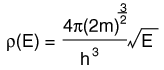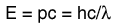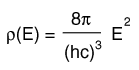The Distribution of Energy

The distribution of a fixed amount of energy among a number of identical particles depends upon the density of available energy states and the probability that a given state will be occupied. The probability that a given energy state will be occupied is given by the distribution function, but if there are more available energy states in a given energy interval, then that will give a greater weight to the probability for that energy interval.The distribution function The density of states
Index

Applied statistics concepts

 HyperPhysics***** Quantum Physics R Nave
Go Back

The Density of States

The distribution of energy between identical particles depends in part upon how many available states there are in a given energy interval. This density of states as a function of energy gives the number of states per unit volume in an energy interval. The term "statistical weight" is sometimes used synonymously, particularly in situations where the available states are discrete. The physical constraints on the particles determine the form of the density of states function.

For electrons in a metal the density of states comes from the wave nature of the electron in the "particle in a box" type setting.Show
For photons where the relationship between energy and wavelength isthe density of states isShow
 Distribution of energy
Index

Applied statistics concepts

 HyperPhysics***** Quantum Physics R Nave
Go Back

Distinguishability of Particles

The Einstein-Bose and Fermi-Dirac distributions differ from the classical Maxwell-Boltzmann distribution because the particles they describe are indistinguishable.

Particles are considered to be indistinguishable if their wave packets overlap significantly. This kind of consideration comes from the fact that all particles have characteristic wave properties according to the DeBroglie hypothesis. Two particles can be considered to be distinguishable if their separation is large compared to their DeBroglie wavelength.

For example, the condition of distinguishability is met by molecules in an ideal gas under ordinary conditions. For oxygen gas at STP, the molecules have a separation on the order of 3 nm and DeBroglie wavelengths on the order of 0.03 nm, a factor of a hundred smaller. On the other hand, two electrons in the first shell of an atom are inherently indistinguishable because of the large overlap of their wavefunctions.

Index

Reference
Rohlf
Sec. 12-1

 HyperPhysics***** Quantum Physics R Nave
Go Back